This tutorial includes code and excersizes used in the 2018 Analytical Paleobiology Course, hosted at University of Florida.

Code and datasets associated with this tutorial are available at https://github.com/katrinajones/qpal

These can be installed with `devtools` using `install_github("katrinajones/qpal", build_vignette=T)`

# Introduction to Geometric Morphometrics

See Lecture one in accompanying PDFs.

Geometric morphometrics (GMM) is the quantitative representation and analysis of morphological shape using geometric coordinates instead of measurements.

The goal of morphometrics is to measure morphological similarity and difference. Key features of GMM are:

• Shape is represented by landmarks
• Variables are cartesian coordinates
• must be registered with procrustes analysis
• coordinate system has no objective scale - size not included
• results can be visualized as pictures
• there are several variants on the ‘standard’ analysis e.g., EDMA

Steps in a GMM analysis are:

• Collect landmark data
• Do a Procrustes superimposition
• Analyze similarity and difference of shape

### Collecting landmarks

1. Each shape must have the same number of landmarks
2. The landmarks on all shapes must be in the same order
3. Landmarks are ordinarily placed on homologous points, points that can be replicated from object to object based on common morphology, common function, or common geometry.

### Procrustes superimposition

Procrustes superimposition is the ‘standardization’ step in GMM. Procrustes removes size, translation, and rotation. In orther words, it centers the shapes on the same point, scales them to the same size, and rotates them into the same orientation. These manipulations remove statistical degrees of freedom, which has implications for later statistical analyses. After landmarks have been superimposed, the similarities and differences in their shape can be analyzed.

Other names for procrustes superimposition include: Procrustes analysis, procrustes fitting, generalized procrustes analysis (GPA), generalized least squares (GLS), least squares fitting. The analysis centers the shape on the origin, scales all shape to unite size, and minimizes least squares distance by rotation.

### Principal components analysis (PCA)

PCA ordinates the objects by arranging them in a shape space. Similarities and differences can be easily seen in a PCA plot.

The axes of a PCA plot are principal components (PCs). The first PC is, by definition, the line that spans the largest axis of variation in shape. The second spans the next largest axis of variation at right angles to the first, the third spans the third largest axis of variation, and so on.

The PCA plot is a morphospace:

• each point represents a unique shape
• a shape model can be constructed for every point
• shape forms a continuous gradient through the PC space
• Each PC axis describes a different component of shape
• Real shapes are found where their landmarks correspond to the shape space
• Coordinates of the shapes are scores
• Each exis is orthogonal or uncorrelates
• Each axis accounts for a descending proportion of the total shape variance
• Positive/negative directions of PC axes are entirely arbitrary
• Axes are scaled in Procrustes units (meaningless except within-study)

Morphospace is always centered on the average shape, or consensus shape.

Why is PCA a standard step?

• Rotates data to its major axes for better visualization
• Preserves original distances between points, in other words it does not distort the variation in the data, but only if the covariance matrix is used (standard in GMM)
• Removed correlations between landmark coordinates and adjusts to proper degrees of freedom to simplify statistical analyses.

### Visualization

Thin plate splines are a visualization tool which involves deforming a uniform grid to show a difference between two objects.

Lollipops show vector from each landmark in one shape to another, to visualize shape changes.

### Definitions

Landmarks: ant point described by cartesian coordinates, used to represent the shape of a structure.

Landmarks (2): any point that can be places on a biologically or geometrically homologous point on the structure.

Semi-landmark: a point that is placed arbitrarily using an algorithm, often by defining endpoints at biologically homologous points and placing a specified number of semilandmarks between them.

Sliding semi-landmarks: semilandmarks points whose positions are algorithmically adjusted to minimize either the Procrustes distance or ‘bending energy’. However, placement is sample dependent.

Surfaces: semilandmark representatikon of the 3D surface of an object. Semilandmarks are quantified as cartesian coordinates. Either ordinary (object-dependent) or sliding (sample dependent) semilandmarks.

Consensus shape: the mean of the procrustes coordinates, landmark by landmark

Centroid: the geometric center of the object (the average of all of the x/y/z)

Procrustes distance: the sum of all the distances betweent the corresponding landmarks of two shapes. The main measure of shape difference.

Centroid size: Sum of distances from landmarks to centroid, measure of overall size.

1. Results can be visually represented as shapes
2. Data are easily collected from digital photographs
3. Size is mathematically removed from the analysis to focus on pure shape

1. Size is absent from analysis, and size may be biologically relevant
2. Only single rigid structures can be easily analyzed
3. GMM cannot measure variation in a single landmark. Procrustes distributes variation by least-squares to minimize differences between whole shapes. Variation at an individual landmark cannot be interpreted as biological variation. Use EDMA instead.

### How do you choose landmarks?

1. Data must reflect a hypothesis
2. Data must represent the shape adequately
3. Landmarks must be present on all specimens
4. Measurement error always exists in any collection of data, but ME doesn’t matter if its substantially less than the differences you want to measure.
5. Sample size required for a particular study depends on the within-group variation relative to differences between groups.

### How many specimens do I need?

• Depends on the question!
• Depends on the error in the data
• You need more specimens when the differences you want to measure are small compared to the variation within your group

### What morphometrics can NOT tell you

1. Morphometrics does not tell you what ‘large’ or ‘difference’ or ‘shape’ mean.
2. Morphometrics does not tell you wether you unwittingly have two unrecognizes groups in a single sample
3. How to identify cladistic characters.

## Introduction to Geomorph Package

See Lecture two in accompanying PDFs.

Geomorph provides a flexible tool for analyzing geometric morphometric data. For more information see `?(geomorph)`.

``````#load geomorph
library(geomorph, quietly=T)
library(qpal, quietly=T) #package including functions for the course

#Get example data - plethodon salamander heads

#What's included in the dataset
summary(plethodon)
#>         Length Class  Mode
#> land     960   -none- numeric
#> species   40   factor numeric
#> site      40   factor numeric
#> outline 7262   -none- numeric``````

The ‘wide’ data format (n(pk)) for data is most common outside GMM packages, however, within GMM packages the long format (pkn, 3D array) is most commonly used.

Converting between wide and long data formats:

``````#Converting between wide and long formats
#Long to wide
wide<-two.d.array(plethodon\$land)
wide[1:5,1:5]
#>           [,1]     [,2]      [,3]     [,4]     [,5]
#> [1,]  8.893720 53.77644  9.268400 52.77072 5.561040
#> [2,]  8.679762 54.57819  8.935628 53.83027 5.451914
#> [3,]  9.805328 56.06903 10.137712 55.27961 6.647680
#> [4,]  9.637164 58.03294  9.952104 56.77318 6.109836
#> [5,] 11.035692 58.75009 11.335110 57.85184 8.255382
#Wide to long
long<-arrayspecs(wide, 12, 2)
long[,,1]
#>           [,1]     [,2]
#>  [1,]  8.89372 53.77644
#>  [2,]  9.26840 52.77072
#>  [3,]  5.56104 54.21028
#>  [4,]  1.87340 52.75100
#>  [5,]  1.28180 53.18484
#>  [6,]  1.24236 53.32288
#>  [7,]  0.84796 54.70328
#>  [8,]  3.35240 55.76816
#>  [9,]  6.29068 55.70900
#> [10,]  8.87400 55.25544
#> [11,] 10.74740 55.43292
#> [12,] 14.39560 52.75100``````

Arrays are much more handy for handling GMM data in R because you can easily access all elements with simple code

``````#Indexing arrays
#Get first specimen
long[,,1]
#Get first landmark of all specimens
long[1,,]
#Get X coordinates of all landmarks
long[,1,]``````

Geomorph data frames are a useful way of tidying all your data associated with your landmarks together into a single object, and are the native and sometimes required format for geomorph functions

``````#Create geomorph data frame
plethgdf<-geomorph.data.frame(landmarks=long, species=plethodon\$species,
site=plethodon\$site)
summary(plethgdf)
#>           Length Class  Mode
#> landmarks 960    -none- numeric
#> species    40    factor numeric
#> site       40    factor numeric``````

Once your data is all combined into a single geomorph data frame, its very useful to be able to subset it all at once, keeping track of all your separate variables. I’ve included a custom subsetting function I wrote for this purpose.

``````#plot first five specimens
plot(plethgdf\$landmarks[,,1])

#plot raw data together
plotAllSpecimens(plethgdf\$landmarks, mean=F)``````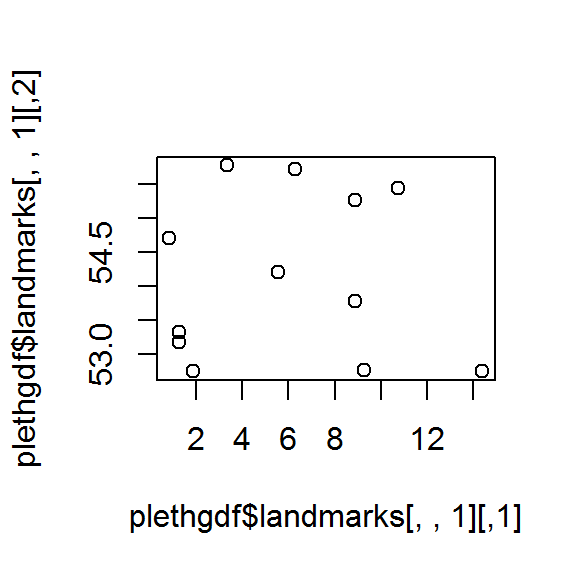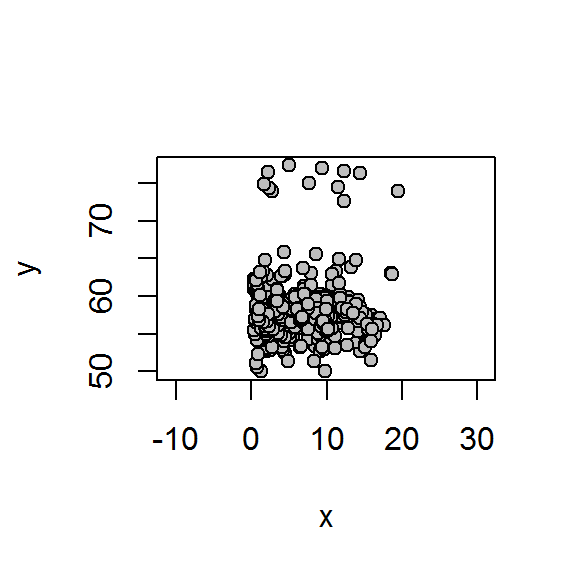``````#plot in same shape space
Pcoords<-gpagen(plethgdf\$landmarks)
plethgdf<-geomorph.data.frame(plethgdf, coords=Pcoords\$coords,
size=Pcoords\$Csize)``````
``plotAllSpecimens(plethgdf\$coords, mean=T, links=plethodon\$links)``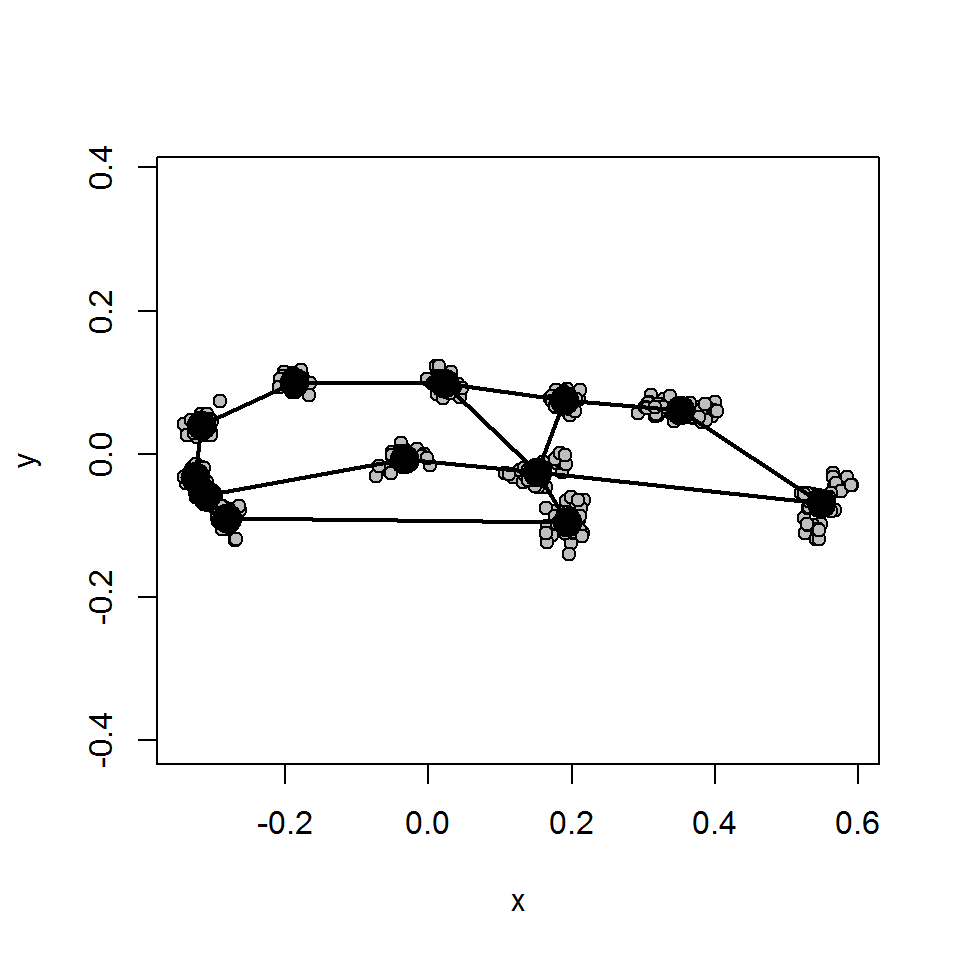Comparing specimens

``````#Are there any wierd specimens
plotOutliers(plethgdf\$coords)``````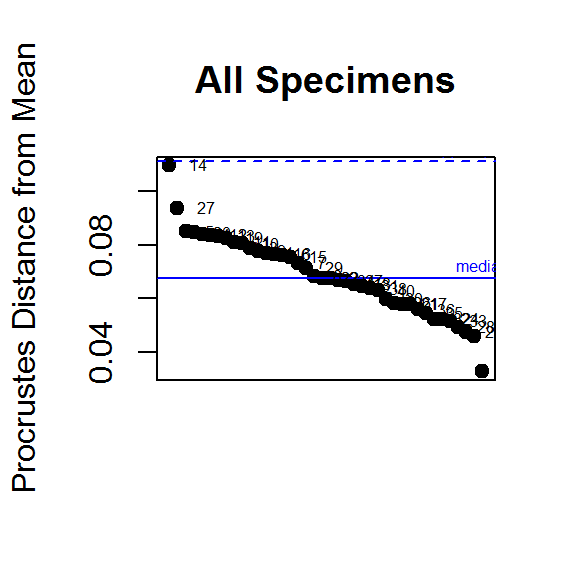``````#Let's compare them
shape1<-mshape(plethgdf\$coords)#consensus shape
shape2<-plethgdf\$coords[,,14]

#Thin plate spline
plotRefToTarget(shape1, shape2, method=c("TPS"), mag=2)
#Lollipops
plotRefToTarget(shape1, shape2, method=c("vector"), mag=10)
#Points
plotRefToTarget(shape1, shape2, method=c("points"), mag=10)``````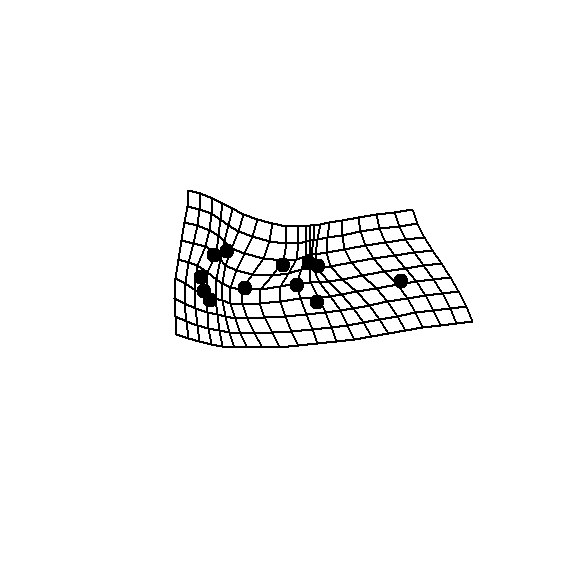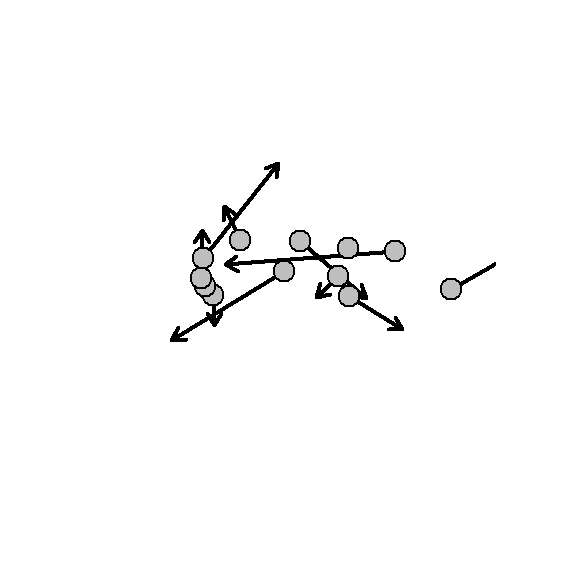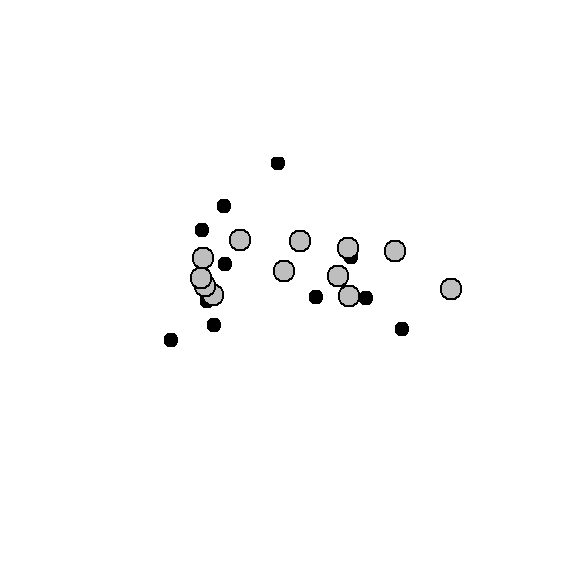# Overview: Your first GMM analysis

## Step-by-step morphometric analysis

This section provides a quick overview of the steps of a GMM analysis in R, which will be followed by a practical in which you will collect and run your own data.

The R package Stereomorph provides a nice interface for collecting landmarks and curves in 2D. You must have either Chrome or Safari installed for this to work. For more guidence on collecting landmarks in Stereomorph see https://aaronolsen.github.io/software/stereomorph/StereoMorph%202D%20Tutorial.pdf

``````library(StereoMorph)
#> Warning: package 'StereoMorph' was built under R version 3.4.4
library(abind)

#Digitize 2D landmarks with Stereomorph
extdatafiles <- system.file("extdata", package="qpal")#find directory where external data are saved
setwd(extdatafiles)``````
``````#Name landmarks
lands<-c("condyle", "angular", "coronoid", "posterior molar", "tip incisor")
#Name curves
curves<-matrix(c("condtocor", "condtoang","condyle","condyle","coronoid",
"angular"), nrow=2,ncol=3)
#Digitize specimens
digitizeImage(image.file = 'ShrewsAndMarmots', shapes.file = 'teeth',
landmarks.ref = lands, curves.ref = curves)``````

``````
#Look at data based on fixed landmarks only
``````
fixed<-teeth\$landmarks.scaled
#Procrustes fit
gpa.lands <- gpagen(fixed)``````
``````plotAllSpecimens(fixed)
plot(gpa.lands)``````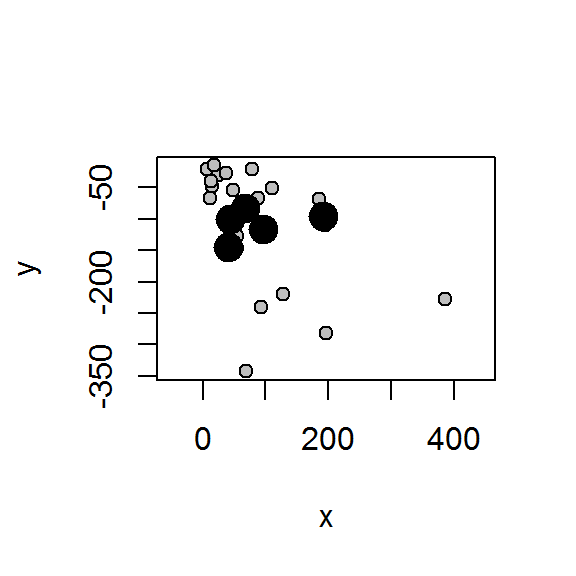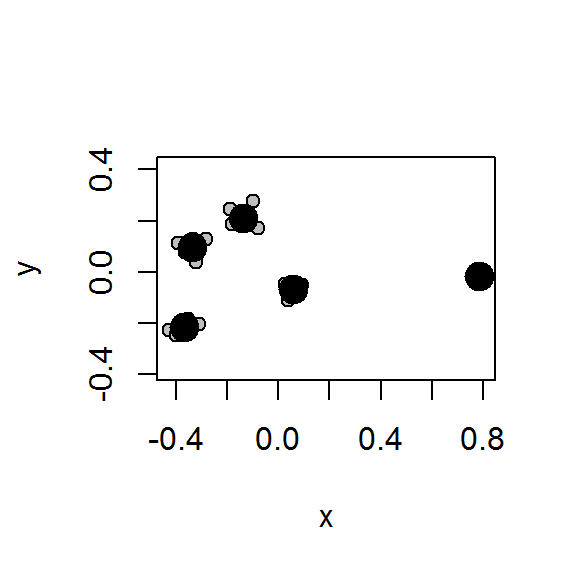`````` #With Sliding semi-landmarks
curves<-teeth\$curves.scaled

#resample curves to 5 fixed landmarks
nfixed<-5
totfixed<-nfixed+2
curvelands<-list()
curvelands<-lapply(curves, function (x) lapply(x, pointsAtEvenSpacing, n=totfixed))#Resample
curvelands<-lapply(curvelands, do.call, what=rbind)#merge
curvelands<-array(unlist(curvelands), dim=c((2*totfixed),2,5))#convert to array

#The first and last points are the original fixed landmarks, so can be dropped
curvelands<-curvelands[-c(1,totfixed, (totfixed+1),(2*totfixed)),,]#remove fixed points
rownames(curvelands)<-rep(c("cu1", "cu2"), each=nfixed) #label
lands<-abind::abind(fixed, curvelands, along=1) #join with fixed``````

We can write to TPS format for use in other software, or read in files created elsewhere in that format

``````#Read and write TPS file for use in other software
writeland.tps(lands, "shrewtest.tps")

Now we need to make a ‘sliders’ file to tell geomorph which landmarks to slide during the procrustes fit. Here I’m using custom code `makesliders` which makes the sliders file based on the curve labels

``````#make sliders file
sliders<-makesliders(rownames(lands),id=c("cu1", "cu2"), begin=c(1,1),end=c(3,2))

#Procrustes fit
gpa.lands <- gpagen(lands, curves=sliders)``````
``````plot(gpa.lands\$consensus, col=palette()[as.factor(rownames(lands))], pch=19)
plot(gpa.lands)
plotRefToTarget(gpa.lands\$coords[,,1], gpa.lands\$consensus)``````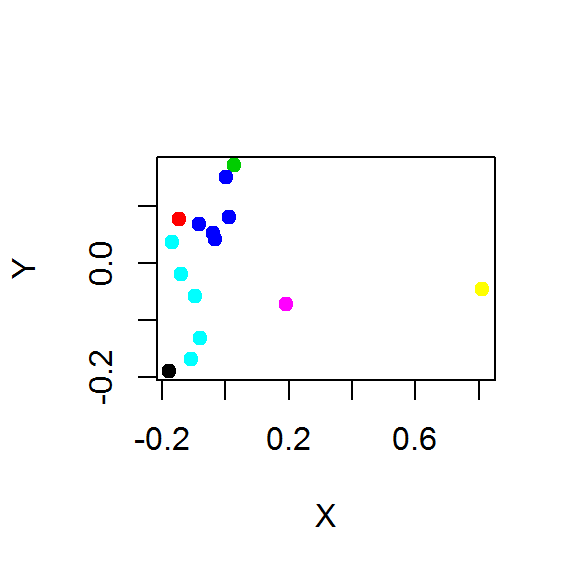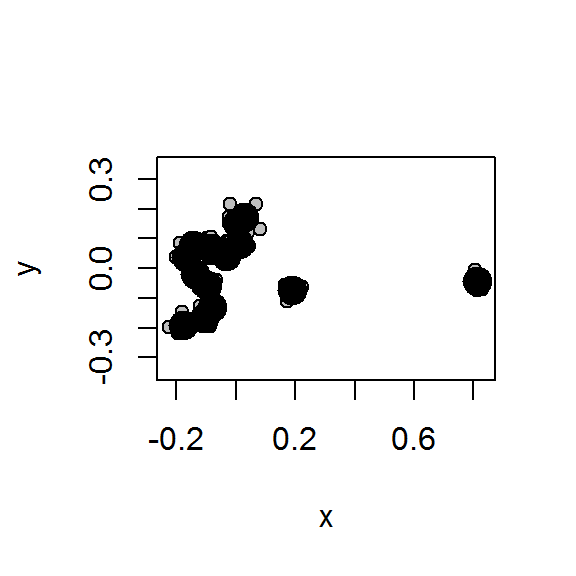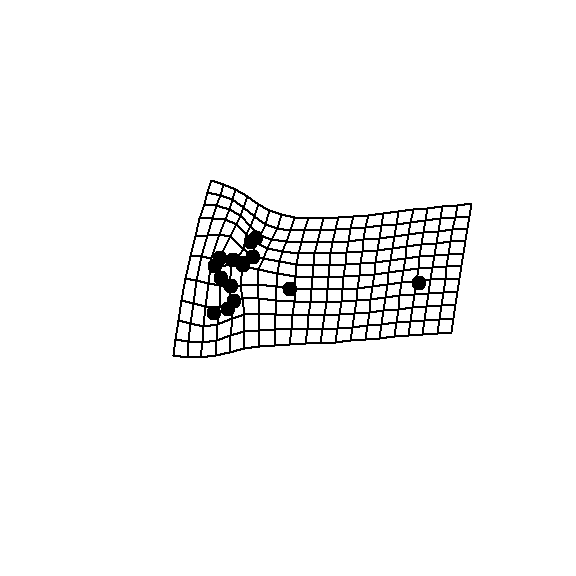Now we’ll try with a much bigger dataset, see `?shrewteeth`

``````##Now lets try with a big dataset of shrewteeth!
#shrew teeth
data("shrewteeth")
summary(shrewteeth)
#>    Min. 1st Qu.  Median    Mean 3rd Qu.    Max.
#>    60.0   314.0   442.0   450.1   555.0   891.0``````
``````#Procrustes fit
proc<-gpagen(shrewteeth)``````
``````#Plot raw data
plotAllSpecimens(shrewteeth)
#plot procrustes coordinates
plot(proc)``````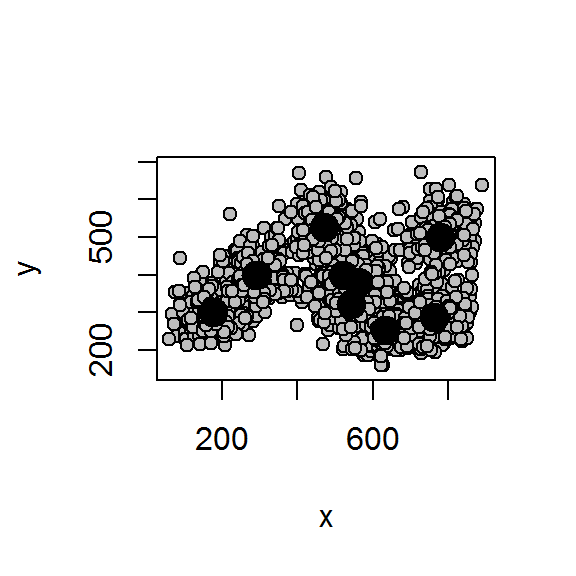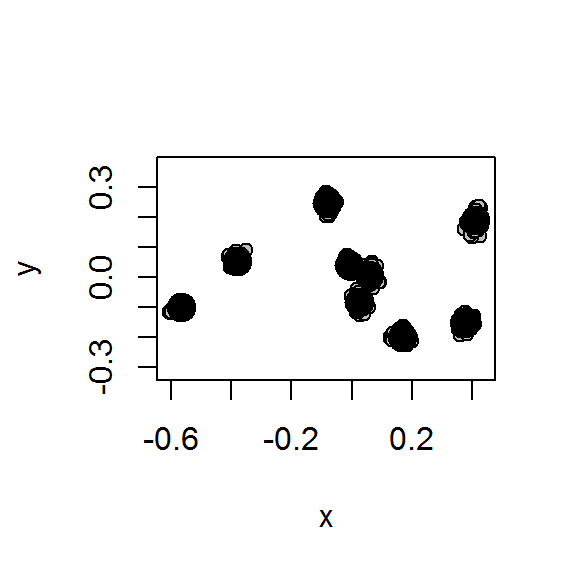Now lets try putting it in a geomorph data frame

``````#Make geomorph data frame
group<-as.factor(rep(c("a", "b"), length=dim(shrewteeth)[]))#make up some labels
shrewdf<-geomorph.data.frame(raw=shrewteeth, coords=proc\$coords,
size=proc\$Csize, group=group)

#subsample by labels
a<-subsetgeom(shrewdf, "spec", which(shrewdf\$group=="a"), keep=T)

#PCA
pca.lands <- plotTangentSpace(shrewdf\$coords, label=TRUE,
groups = palette()[shrewdf\$group])``````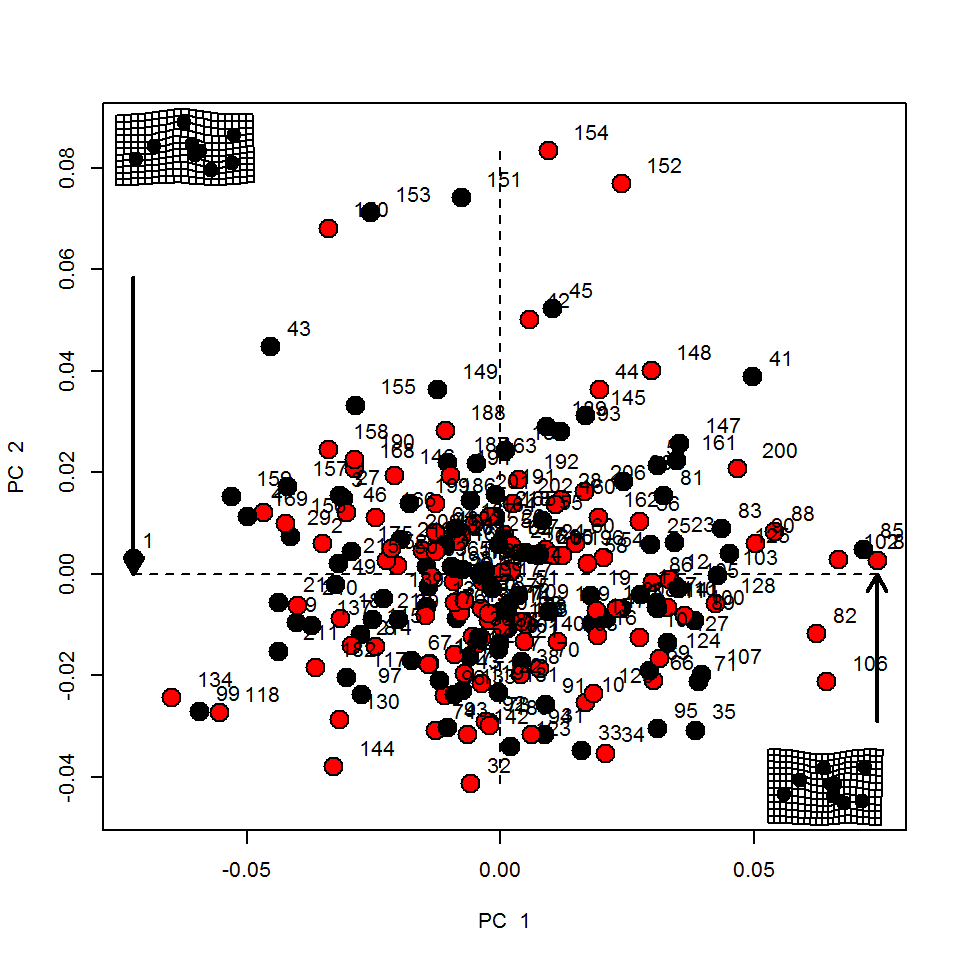``shrewdf<-geomorph.data.frame(shrewdf, scores=pca.lands\$pc.scores)``

Now lets try an interactive 3D plot

``````plot3d(shrewdf\$scores[,1:3])#interactive 3D plots
text3d(shrewdf\$scores[,1:3],texts=rownames(shrewdf\$scores),pos=4,cex=.5)``````